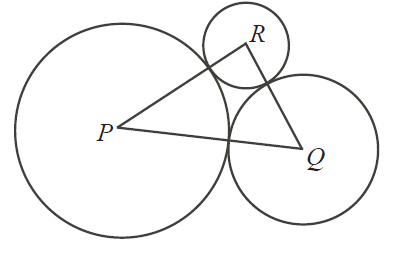# Area of Triangle PQR

Geometry Level 2In the diagram, the circles with centers $P, Q, R$ have radii $3, 2, \text{ and} \ 1$ respectively. Each circle touches the other two as shown. What is the area of triangle $PQR$?

×